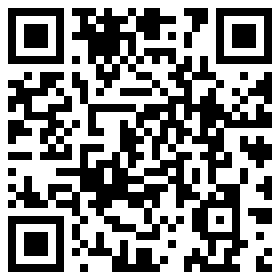| | |

|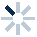|
APP端下载AndroidiPhone
|
￥42

|
21人点赞
46238人已学习
|

3天无理由退款

3天无理由退款：退款将以超级币形式退至您的超级课堂学习账户，便于您重新选购其他课程。恶意退款将被冻结账号。

• 1、掌握物体浸于分层液体之间的规律：物体受到的浮力大小等于它排开所有液体的总重力
2、 若物体浸于两种分层液体之中，则由$G=F_{浮1}+F_{浮2}$可得：$ρ_{物}gV_{物}=ρ_{1}gV_{1}+ρ_{2}gV_{2}$，即$ρ_{物}V_{物}=ρ_{1}V_{1}+ρ_{2}V_{2}$
• 1、介绍物体浸于分层液体之间的特殊情况，即悬浮于两层液体之间的两个条件：(1)两层液体都必须足够多；(2)物体的密度必须介于两层液体密度之间，即$ρ_{1}＜ρ_{物}＜ρ_{2}$
2、 介绍物体悬浮于两层液体之间时上下浸入体积之比，$\dfrac{V_{1}}{V_{2}}=\dfrac{ρ_{2}-ρ_{物}}{ρ_{物}-ρ_{1}}$，即物体与哪层液体密度更接近，则物体在哪层液体中浸入得更多；若物体密度恰好等于两种液体密度的平均值，$ρ_{物}=\dfrac{ρ_{1}+ρ_{2}}{2}$，则物体在两层液体中浸入体积相等
• 1、介绍运用浮力法测液体密度的前三种方法：第一种是最简单的密度计法，使密度计竖直漂浮在待测液体中，根据液面在密度计上的位置就可以直接读出液体的密度
2、 第二种是阿基米德原理法，原理就是$ρ_{液}=\dfrac{F_{浮}}{gV_{排}}$
3、 第三种是悬提法，原理是：在调平的天平左盘烧杯中浸没固体时，天平右盘增加的砝码重力等于固体受到的浮力，$F_{浮}=\Delta mg$
• 1、介绍运用浮力法测液体密度的后两种方法：第四种是双漂法，将同一个密度较小的固体分别漂浮在密度已知的液体（通常是水）和待测液体中，由等浮力原理推导得$ρ{液}=\dfrac{V_{排1}}{V_{排2}}ρ_{0}$
2、 第五种是双沉法，将同一个密度较大的固体分别浸没在密度已知的液体（通常是水）和待测液体中（不与容器底接触），由等体积原理推导得$ρ_{液}=\dfrac{G-F_{2}}{G-F_{1}}ρ_{0}$
• 1、介绍了利用浮力法测固体密度的前三种方法：第一种方法是悬浮测液法，是一种间接测量固体密度的方法，一般用来测量密度比水大但大得不多的固体密度。将物体浸没在水中，加盐直到物体悬浮在盐水中，取出物体，用密度计测出盐水的密度，即物体的密度：第二种方法是曹冲称象法，也叫等效替代法，质量等于使小烧杯下沉同样深度的水的质量，体积可以用液面差法来测出：第三种方法是溢水法，原理是：若物体在液体中漂浮或悬浮，可以由溢水法直接得到物体的质量；而若物体在液体中悬浮或下沉，可以由溢水法直接得到物体的体积。所以只利用量筒就能测出密度小于水的物体密度
• 1、介绍利用浮力测固体密度的方法的另外三种方法：第四种方法是两提法，用于测密度大于水的固体密度，先用弹簧测力计测出待测物体的重力$G$，再将待测物体浸没在水中，读出弹簧测力计的示数$F$，则物体的密度$ρ=\dfrac{G}{G-F}ρ_{水}$
2、 第五种方法是悬提法，先用天平测出待测物体的质量$m_{1}$、装有适量水的烧杯的质量m_{2}，再用细线系上待测物体放入装水的烧杯中，且不与烧杯底接触，调节天平平衡记下m_{3}。物体的密度$ρ=\dfrac{m_{1}}{m_{3}-m_{2}}ρ_{水}$
3、 第六种方法是漂压法，通常用于测量密度小于水的物体密度。分别测出水的体积$V_{1}$、待测物体漂浮于水中时的体积$V_{2}$、将待测物体压入水中时的体积$V_{3}$，则物体的密度$ρ=\dfrac{m}{V}=\dfrac{V_{2}-V_{1}}{V_{3}-V_{1}}ρ_{水}$
4、 对于与小船类似的可以漂浮也可以沉底的物体，或者橡皮泥这种可以改变形状的物体，即使密度大于水，也可以先漂浮得到质量，再沉底得到体积，最后计算出密度
• 1、介绍利用浮力测固体密度的第七种方法：一漂一沉法，它用来测量密度大于水，且无法改变形状的物体。公式与漂压法相同，都是$ρ=\dfrac{V_{2}-V_{1}}{V_{3}-V_{1}}ρ_{水}$，这里的$V_{1}$、$V_{2}$、$V_{3}$分别指只放入漂浮物时的体积值、待测物体放在漂浮物内部或上方时的体积值、将待测物体单独沉入水中时的体积值
2、 通过两个实例介绍了两种特殊的一漂一沉法，第一种是如果没有量筒的情况，可以借助形状规则的漂浮物，通过测量漂浮物露出水面的长度来间接得到浸入水中的体积；第二种是有量筒但口径不足，无法直接将待测物体放入其中来测量，可以再借助溢水法来操作
• 浮力的计算与综合应用下综合练习

• 1超级学员840452
• 2海水有点甜
• 3超级学员3297891
• 4超级学员3795558
• 5超级学员3919003
• 6花谢花飞花
• 7超级学员3954473
• 8超级学员3957174
• 9超级学员3959428
• 10超级学员4213287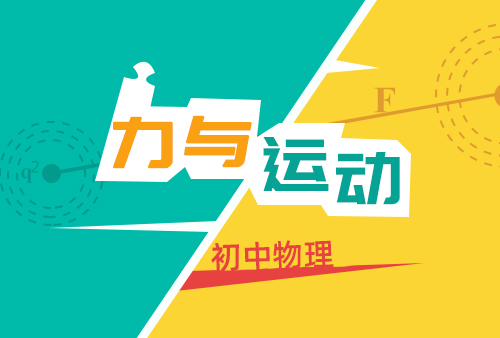206285人在学
￥ 78 ￥ 78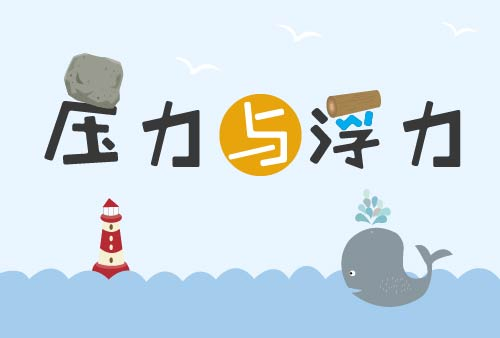204850人在学
￥ 32 ￥ 32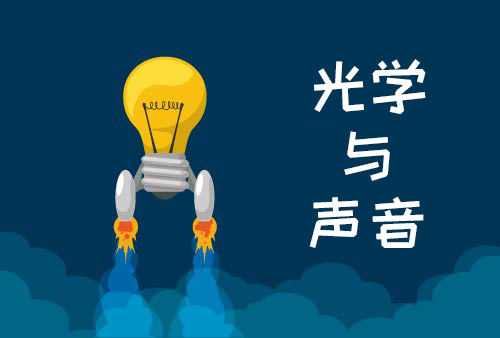206361人在学
￥ 64 ￥ 64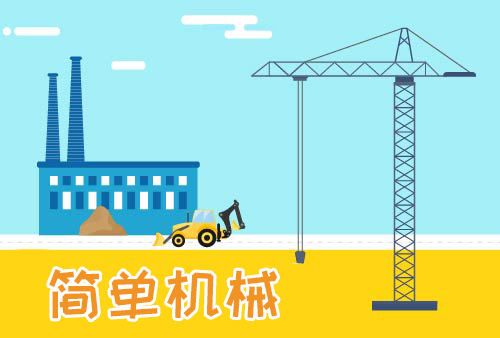205676人在学
￥ 32 ￥ 32

• 0
• 点击分享有好礼
•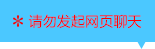•app端下载关注微信号